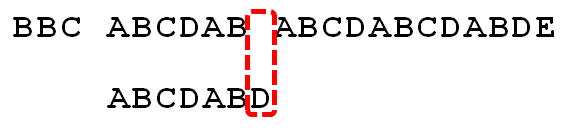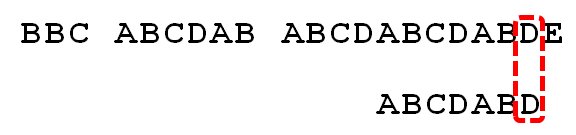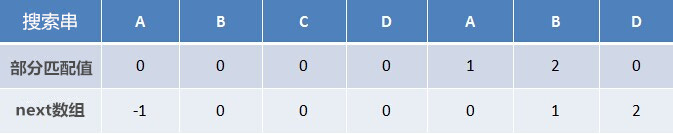[算法系列之二十六]字符串匹配之KMP算法

KMP算法是一种改进的字符串匹配算法，由D.E.Knuth与V.R.Pratt和J.H.Morris同时发现，因此人们称它为克努特—莫里斯—普拉特操作（简称KMP算法）。KMP算法的关键是利用匹配失败后的信息，尽量减少模式串与主串的匹配次数以达到快速匹配的目的。移动位数 = 已匹配的字符数 - 失配字符的上一位字符对应的部分匹配值• 前缀指除了最后一个字符以外，一个字符串的全部头部组合；
• 后缀指除了第一个字符以外，一个字符串的全部尾部组合。“部分匹配”的实质是，有时候，字符串头部和尾部会有重复。比如，”ABCDAB”之中有两个AB，那么它的”部分匹配值”就是2（AB的长度）。搜索词移动的时候，第一个AB向后移动4位（字符串长度-部分匹配值），就可以来到第二个AB的位置。

4.1：根据《部分匹配表》求next 数组移动位数 = 已匹配的字符数 - 失配字符的上一位字符对应的部分匹配值移动位数 = 失配字符的位置 - 失配字符next值


4.2 递推求解next数组

• 如果p[k] = p[j]，则p[0…k]=p[j-k…j]，所next[j+1]=k+1=next[j]+1
• 如果p[k] != p[j]，这是可以看做另外一个字符串匹配的问题，主串和模式串都是p，当匹配失败时，k应该如何移动呢？显然是k=next[k]
    void GetNext(string T,int next[]){
int size = T.size();
next = -1;
int k = -1;
int j = 0;
while(j < size - 1){
// p[k]表示前缀，p[j]表示后缀
if(k == -1 || T[k] == T[j]){
++k;
++j;
next[j] = k;
}//if
else{
// 回溯
k = next[k];
}
}//while
}